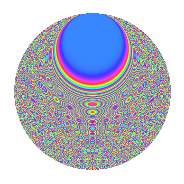# Properties

 Label 1008.2.dyLevel 1008 Weight 2 Character orbit dy Rep. character $$\chi_{1008}(173,\cdot)$$ Character field $$\Q(\zeta_{12})$$ Dimension 752 Sturm bound 384

# Related objects

## Defining parameters

 Level: $$N$$ = $$1008 = 2^{4} \cdot 3^{2} \cdot 7$$ Weight: $$k$$ = $$2$$ Character orbit: $$[\chi]$$ = 1008.dy (of order $$12$$ and degree $$4$$) Character conductor: $$\operatorname{cond}(\chi)$$ = $$1008$$ Character field: $$\Q(\zeta_{12})$$ Sturm bound: $$384$$

## Dimensions

The following table gives the dimensions of various subspaces of $$M_{2}(1008, [\chi])$$.

Total New Old
Modular forms 784 784 0
Cusp forms 752 752 0
Eisenstein series 32 32 0

## Trace form

 $$752q - 6q^{2} - 6q^{3} + 2q^{4} - 12q^{5} + O(q^{10})$$ $$752q - 6q^{2} - 6q^{3} + 2q^{4} - 12q^{5} - 12q^{10} - 6q^{12} - 6q^{14} - 16q^{15} + 2q^{16} + 26q^{18} - 12q^{19} - 16q^{21} - 4q^{22} - 6q^{24} - 12q^{29} - 50q^{30} - 12q^{31} - 6q^{32} - 12q^{33} - 12q^{34} + 30q^{35} + 20q^{36} - 4q^{37} - 12q^{38} + 18q^{42} - 4q^{43} + 66q^{44} - 6q^{45} - 12q^{46} - 12q^{47} - 4q^{49} - 12q^{50} + 50q^{51} - 90q^{54} - 48q^{56} - 4q^{58} - 6q^{59} + 6q^{60} - 6q^{61} - 24q^{62} - 36q^{63} - 16q^{64} - 12q^{65} - 6q^{66} + 2q^{67} - 12q^{68} - 18q^{69} - 16q^{70} - 46q^{72} - 36q^{75} - 6q^{77} + 26q^{78} + 4q^{79} - 4q^{81} - 24q^{82} - 60q^{83} - 24q^{84} + 6q^{85} - 4q^{88} + 108q^{90} + 20q^{91} - 12q^{92} - 14q^{93} - 6q^{94} - 12q^{95} - 108q^{96} + 90q^{98} - 26q^{99} + O(q^{100})$$

## Decomposition of $$S_{2}^{\mathrm{new}}(1008, [\chi])$$ into newform subspaces

The newforms in this space have not yet been added to the LMFDB.

## Hecke Characteristic Polynomials

There are no characteristic polynomials of Hecke operators in the database